# Flutter - Dart 3α 新特性 Record 和 Patterns 的提前预览讲解

Record 和 Patterns 作为 Dart 3 的 Big Things ，无疑是 Flutter 和 Dart 开发者都十分关注的新特性。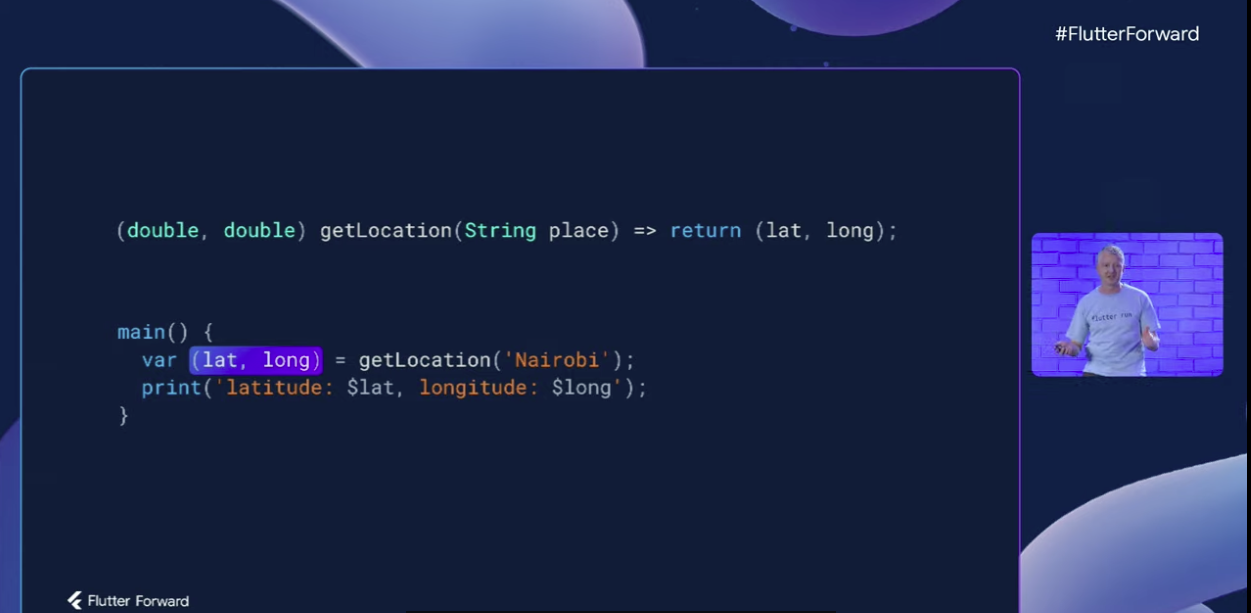# Records

``````var record = (1, a: 2, 3, b: 4);
``````

• 定义了类，也就是说你的数据集合需要和特定类耦合
• 使用 Records 就不必声明对应类型，只要具有相同字段集的记录， Dart 就会认为它们是相同类型（这个后面会介绍）

## 简单介绍

``````  var record = (1, a: 2, 3, b: 4);
print(record.\$1); // Print "1"
print(record.a);  // Print "2"
print(record.\$2); // Print "3"
print(record.b);  // Print "4"
``````

``````  var num = (123);      // num
var records = (123,); // record
``````
• 如果没有 "`,`" ，那么 `(123)` 就是一个 num 类型的对象
• 有 "`,`" 之后 `(123,)` 才会被识别为是一个 Records 类型

``````  (bool, num, {int n, String s}) records;
records = (false, 1, n: 12, s : "xxx");
print(records);
``````

``````  records = (false, 1,  s : "xxx2");
records = (false, 1,  n : 12);
``````

``````  records = (false, 1, s : "xxx2",  n : 12);
records = (s : "xxx2",  n : 12, false, 1, );
print(records);
``````

• 同一命名字段名称只能出现一次，这个不难理解，比如上面代码你不可能定义两个 `s`
• `(,)` 这样的表达式是不允许的，但是 `()` 可以是没有任何字段的常量空 Records
• 有参数但是只有 `()` 没有 "`,`" 也不是 Records ，如 `(6)`
• 命令为 `hashCode``runtimeType``noSuchMethod`, 、`toString` 的字段是不允许的
• 以下划线开头的命令字段是不允许的
• 与位置字段名称冲突的命令字段，比如 `('pos', \$1: 'named')` 这样是不行的，但是 `(\$1: 'records')` 这样可以

``````   var t = (int, String);
print(t);
print(t.\$0.runtimeType);
print(t.\$1.runtimeType);
``````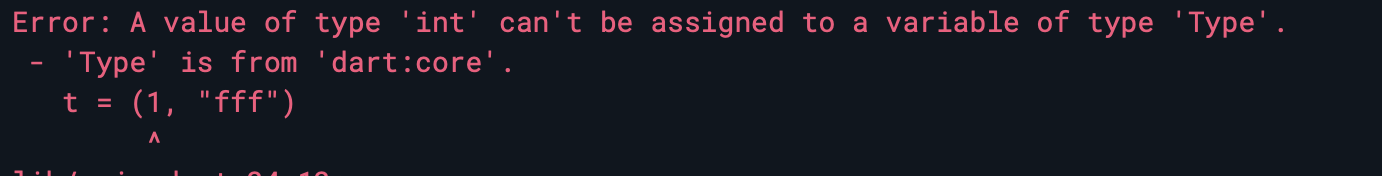• `Record``Object``dynamic` 的子类和 `Never` 的父类
• 所有的 Records 都是 `Record` 的子类和 `Never` 的父类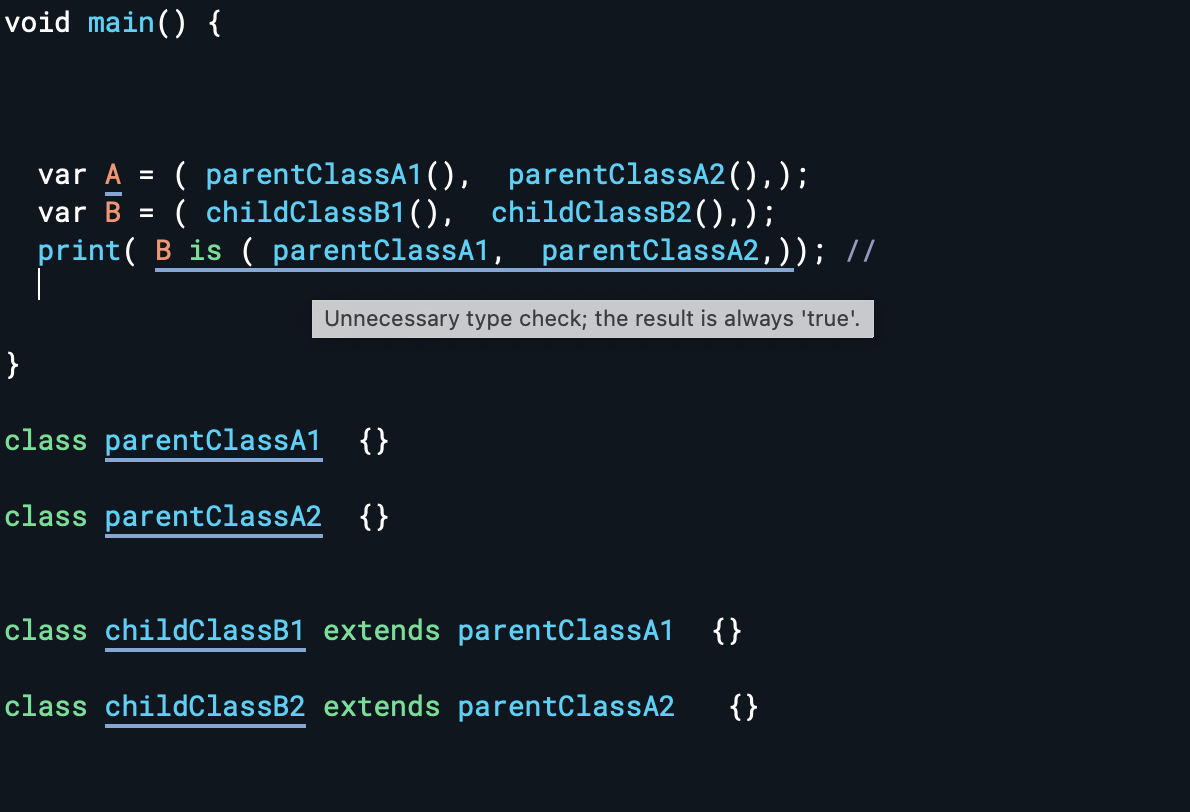## 进阶探索

``````class extends Record {
double get \$1;
String get name;
bool get \$2;
int get count;
}
``````

Records 里每个字段都有 getter ，并且字段是不可变的，所以不会又 Setter

``````print((a: 1, b: 2) == (b: 2, a: 1)); // true
``````

``````print((true, 2, a: 1, b: 2,) == (2, true, b: 2, a: 1)); // false
``````

``````(num, Object) pair = (1, 2.3);
print(pair is (int, double)); // "true".
``````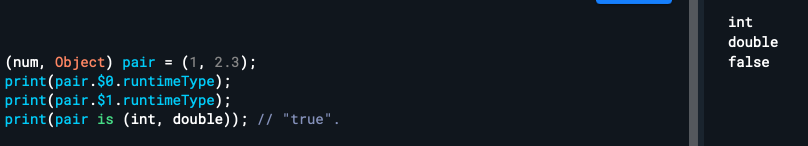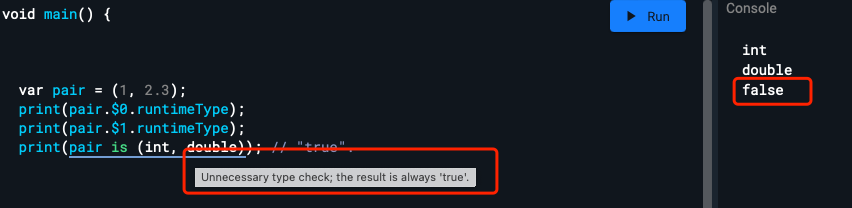``````  var map = {};
map[(1, "aa")] = "value";
print(map[(1, "aa")]); //输出 "value"
``````

``````
class newClass  {

}

var map = {};
map[(1, new newClass())] = "value";
print(map[(1, new newClass())]); //输出 "null"
``````

``````class newClass  {

@override
bool operator ==(Object other) {
return true;
}

@override
int get hashCode => 1111111;

}
``````

``````int say(int i) {
print(i);
return i;
}

var x = (a: say(1), b: say(2));
var y = (b: say(3), a: say(4));
``````

``````var x = (say(0), a: say(1), b: say(2));
var y = (b: say(3), a: say(4), say(5));
``````

## Records 带来的语法歧义

### try/on

``````  void recordTryOn() {
try {
} on String {
}

on(int, String) {
}
}
``````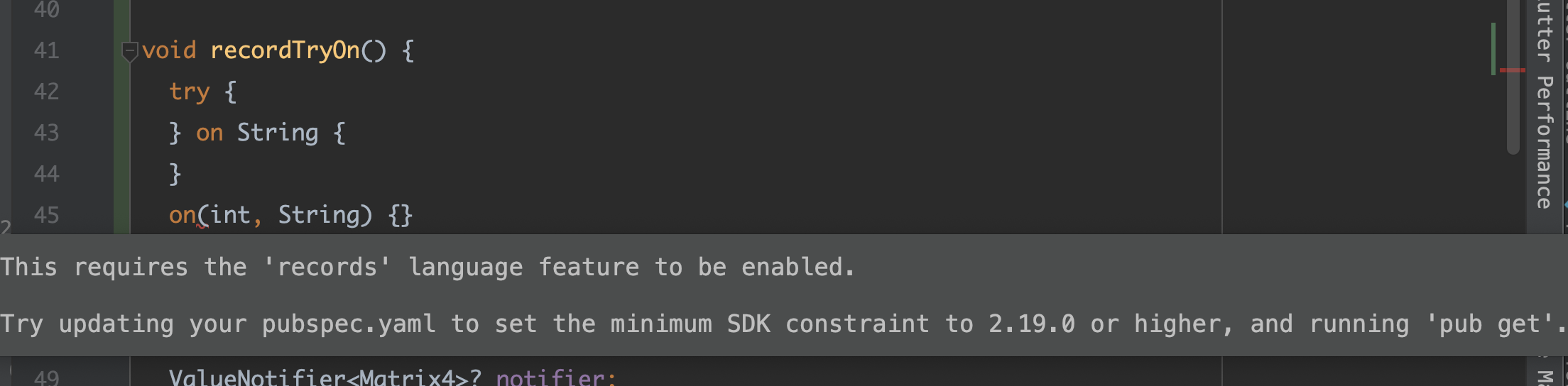``````@metadata (a, b) function() {}
``````

• `@metadata(a, b)` 与没有返回类型的函数声明关联的metadata 注解
• `@metadata`与返回类型为 Records 类型的函数关联的metadata 注解 `(a, b)`

``````@metadata(a, b) function() {}

``````
• 前者由于 `@metadata` 之后没有空格，所以表示为 `(a, b)` 的 metadata 注解
• 前者由于有空格，所以表示为 Records 返回类型

``````//  Records 和 metadata 是一起作用在 a

//  Records 是直接作用在 a ，和 metadata 无关

(x, y) a;

(x,) a;
``````

``````@TestMeta(1, "2")
class C {}

class TestMeta {
final String message;
final num code;

const TestMeta(this.code, this.message);

@override
String toString() => "feature:  \$code, \$message";
}
``````

``````@TestMeta (1, "2") //Error
class C {}
``````

### toString

``````print((1, 2, 3).toString()); // "(1, 2, 3)".
print((a: 'str', 'int').toString()); // "(a: str, int)".
``````

Debug 版本中，命名字段出现的顺序以及它们如何与位置字段进行排列是不确定的，只有位置字段必须按位置顺序出现

# Patterns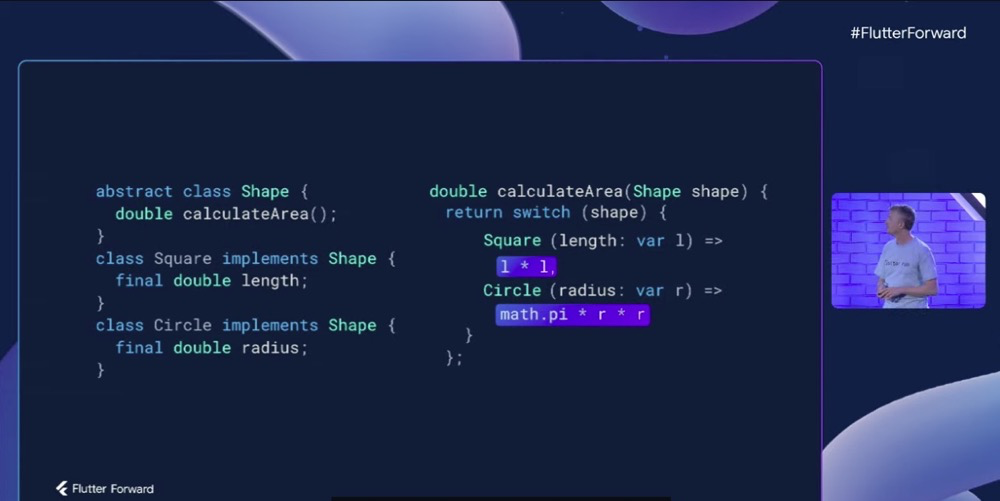## 简单介绍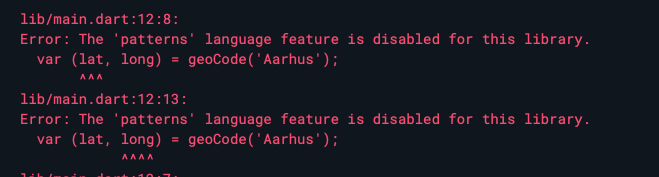### 多返回值

``````(double, double) geoCode(String city) {
var lat = // Calculate...
var long = // Calculate...

return (lat, long); // Wrap in record and return.
}
``````

``````var (lat, long) = geoCode('Aarhus');
print('Location lat:\$lat, long:\$long');
``````

``````var list = [1, 2, 3];
var [a, b, c] = list;
print(a + b + c); // 6.

var map = {'first': 1, 'second': 2};
var {'first': a, 'second': b} = map;
print(a + b); // 3.
``````

``````var (a, b) = ('left', 'right');
(b, a) = (a, b); // Swap!
print('\$a \$b'); // Prints "right left".
``````

### 代数数据类型

``````///before
double calculateArea(Shape shape) {
if (shape is Square) {
return shape.length + shape.length;
} else if (shape is Circle) {
} else {
throw ArgumentError("Unexpected shape.");
}
}

//after
double calculateArea(Shape shape) =>
switch (shape) {
Square(length: var l) => l * l,
Circle(radius: var r) => math.pi * r * r
};
``````

### Patterns

``````var isPrimary = switch (color) {
Color.red || Color.yellow || Color.blue => true,
_ => false
};
``````

`"||"`可以在 switch 中让多个 case 共享一个主体，`"_"` 表示默认，甚至如下代码所示，你还可以在绑定 `s` 之后，多个共享一个 `when` 条件：

``````switch (shape) {
case Square(size: var s) || Circle(size: var s) when s > 0:
print('Non-empty symmetric shape');
case Square() || Circle():
print('Empty symmetric shape');
default:
print('Asymmetric shape');
}
``````

``````String asciiCharType(int char) {
const space = 32;
const zero = 48;
const nine = 57;

return switch (char) {
< space => 'control',
== space => 'space',
> space && < zero => 'punctuation',
>= zero && <= nine => 'digit'
// Etc...
}
}
``````

``````String? maybeString = ...
switch (maybeString) {
case var s?:
// s has type non-nullable String here.
}
``````

``````(int?, int?) position = ...

// We know if we get here that the coordinates should be present:
var (x!, y!) = position;

List<String?> row = ...

// If the first column is 'user', we expect to have a name after it.
switch (row) {
case ['user', var name!]:
// name is a non-nullable string here.
}
``````

``````switch ((1, 2)) {
case (var a, var b): ...
}

switch (record) {
case (int x, String s):
print('First field is int \$x and second is String \$s.');
}
````````````var list = [1, 2, 3];
var [_, two, _] = list;

var [a, b, ...rest, c, d] = [1, 2, 3, 4, 5, 6, 7];
print('\$a \$b \$rest \$c \$d'); // Prints "1 2 [3, 4, 5] 6 7".

// Variable:
var (untyped: untyped, typed: int typed) = ...
var (:untyped, :int typed) = ...

switch (obj) {
case (untyped: var untyped, typed: int typed): ...
case (:var untyped, :int typed): ...
}

// Null-check and null-assert:
switch (obj) {
case (checked: var checked?, asserted: var asserted!): ...
case (:var checked?, :var asserted!): ...
}

// Cast:
var (field: field as int) = ...
var (:field as int) = ...

class Rect {
final double width, height;

Rect(this.width, this.height);
}

display(Object obj) {
switch (obj) {
case Rect(width: var w, height: var h): print('Rect \$w x \$h');
default: print(obj);
}
}
``````